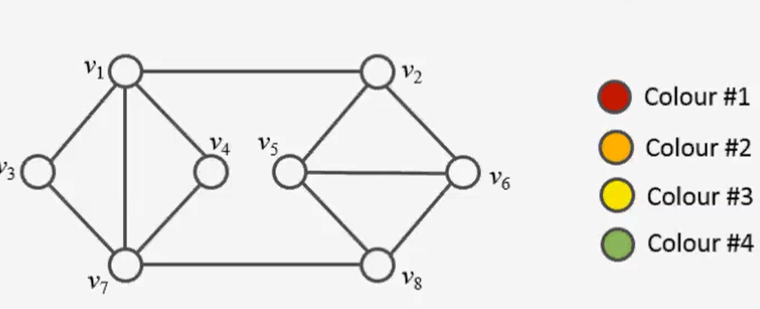## Vertex Coloring using ILPIn this post I’ll show you how to solve the vertex coloring problem to optimality using linear programming. Since the problem is NP-complete, there is no algorithm which can solve any problem instance in deterministic polynomial time. In a former post, I used constraint programming to find an optimal (minimal) vertex coloring. A popular greedy… Read more Vertex Coloring using ILP

## Find optimal Solution to Knapsack Problem with Linear Programming in R

In another post I demonstrated how to develop a heuristic to solve the knapsack problem. We managed to solve the problem quite well and had an optimality gap of about 1%, that is, our solution was 1% away from the optimal solution. Here I’d like to demonstrate, how simple it is to solve the Knapsack… Read more Find optimal Solution to Knapsack Problem with Linear Programming in R

## Calling GUROBI from Matlab

In this post I’ll show you the steps you need to take, if you want to use the powerful GUROBI solver from Matlab in order to solve Mixed Integer Linear Programs. Install the GUROBI Solver Go to the Gurobi website, create an account and download the version applicable for your operating system here. Acquire and… Read more Calling GUROBI from Matlab

## Solve Optimization Tasks using GUROBI CloudWhat is the objective here? I will document the steps needed to setup Gurobi to solve a Linear Program in the cloud. For this example we will use a simple linear model and use Matlab to invoke the locally installed Gurobi solver, which in turn would forward it to a Gurobi Compute Server, which we… Read more Solve Optimization Tasks using GUROBI Cloud